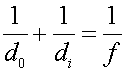Examples for lenses

Example #1

Problem:

a.) A converging lens (convex) has a focal length of 14 cm. Looking through the lens, one sees an image 20 cm behind the lens. Where is the object?

Solution: Since the image is behind the lens, it is virtual and the distance di is negative. Using the formula,, one can solve for do.

do = 8.24 cm

b.) If the height of the object is 1.5 cm, what is the height of the image.

Solution: Using the formula,to get

hi = 3.64 cm, upright

Example #2

Problem:

A real image of a coin is observed 34 cm beyond a lens. The image height is 1.4 cm and it is known that the actual coin is 0.7 cm high. What is the focal length of the lens?

Solution: First, find the object distance using. Remember that since the image is real, that the image height is negative. ( do = 17 cm ). One can then useto find the focal length.

f = 11.33 cm

Example #3

Problem:

An object is place half way between a converging lens and it's focal point. The focal distance is 8 cm.

a.) Where is the image?

Solution: Usewith 4 cm for do.

di = -8 cm

b.) What is the magnification?

m = 2

Lenses index        Lecture index